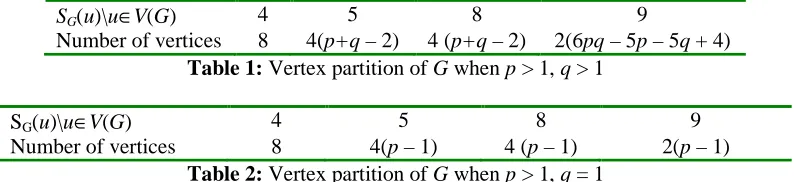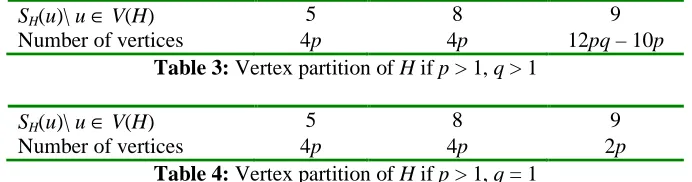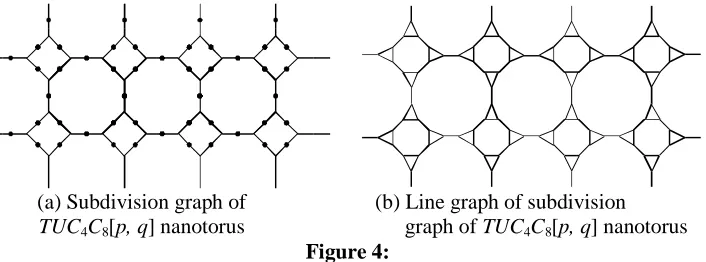# Multiplicative Neighborhood Indices

N/A
N/A
Protected

Share "Multiplicative Neighborhood Indices"

Copied!
7
2
0

Full text

(1)

Vol.19, No.2, 2019, 175-181

ISSN: 2279-087X (P), 2279-0888(online) Published on 20 April 2019

www.researchmathsci.org

DOI: http://dx.doi.org/10.22457/apam.614v19n2a6

175

Annals of

## Multiplicative Neighborhood Indices

V.R. Kulli

Department of Mathematics

Gulbarga University, Gulbarga 585106, India e-mail: vrkulli@gmail.com

Received 28 March 2019; accepted 14 April 2019

Abstract. We define the multiplicative total neighborhood index, multiplicative F-neighborhood index and generalized multiplicative version of these indices and determine exact formulas for line graphs of subdivision graphs of 2-D lattice, nanotube and nanotorus of TUC4C8[p, q].

Keywords: Multiplicative neighborhood indices, line graph, subdivision graph, nanostructures.

AMS Mathematics Subject Classification (2010): 05C07, 05C35, 05C90 1. Introduction

Throughout this paper G is a finite, simple, connected graph with vertex set V(G) and edge set E(G). The degree dG(v) of a vertex v is the number of vertices adjacent to v. The

line graph L(G) of G is the graph whose vertex set corresponds to the edges of G such that two vertices of L(G) are adjacent if the corresponding edges of G are adjacent. The subdivision graph S(G) of G is the graph obtained from G by replacing each of its edges by a path of length two. Let NG(v) = {u : uv E(G)}. Let

( ) ( )

( ) G

G G

u N v

S v d u

=

## ∑

be the degree sum of neighbor vertices. For undefined term and notation, we refer to .

We need the following results.

Lemma 1. Let G be a graph with p vertices and q edges. Then S(G) has p+q vertices and 2q edges.

Lemma 2. Let G be a graph with p vertices and q edges. Then L(G) has q vertices and

2 1

1

2 i G i

d u q

=

edges.

(2)

176 ( )

u V G

## ∑

The fifth M1 and M2 Zagreb indices were introduced by Graovac et al. in , defined as

( ) ( ) ( )

( )

1 5 G G ,

uv E G

M G G S u S v

 

=

+ ( ) ( ) ( )

( )

2 5 G G .

uv E G

M G G S u S v

=

## ∑

The fifth multiplicative M1 and M2 Zagreb indices were proposed by Kulli in , defined as

( ) ( ) ( )

( )

1 5 G G ,

uv E G

M G II G S u S v

 

=

+ ( ) ( ) ( )

( )

2 5 G G .

uv E G

M G II G S u S v

=

## ∏

Recently, the fifth arithmetic-geometric index , fourth multiplicative ABC index , fifth multiplicative arithmetic-geometric index , fifth multiplicative hyper Zagreb indices , multiplicative atom bond connectivity index  were introduced and studied.

In , Kulli introduced the multiplicative first neighborhood index, defined as

( ) ( )

( ) 2

1 G .

u V G

NM II G S u

∈ =

## ∏

We introduce the multiplicative total neighborhood index of a graph G, defined as

( ) ( )

( ) .

n G

u V G

T II G S u

=

## ∏

We propose the multiplicative F1-neighborhood index of a graph and it is defined as

( ) ( )

( ) 3

1 G .

u V G

F NII G S u

∈ =

## ∏

We now continue the generalization and define the general first multiplicative neighborhood index of a graph G as

( ) ( ) ( ) 1 a a G u V G

NM II G S u

∈ =

## ∏

(1)

where a is a real number.

In this paper, we compute the multiplicative first neighborhood index, multiplicative total neighborhood index, multiplicative F1-neighborhood index and the general first multiplicative neighborhood index of line graphs of subdivision graphs of 2-D lattice, nanotube and nanotorus of TUC4C8[p, q].

2. 2-D lattice, nanotube and nanotorus of TUC4C8[p, q]

(3)

177

G1 H1 K1

(a) (b) (c)

Figure 1:

(a) 2D-lattice of TUC4C8[4, 2] (b) TUC4C8[4,2] nanotube (c) TUC4C8 [4, 2] nanotorus

3. 2-D lattice of TUC4C8[p, q]

A subdivision graph of 2-D lattice and line graph of subdivision graph of 2-D lattice of TUC4C8[4, 2] are shown in Figure 2(a) and Figure 2(b) respectively.

(a) (b)

(a) subdivision graph of (b) line graph of the subdivision graph 2D-lattice of TUC4C8[4,2] of 2D-lattice of TUC4C8 [4, 2]

Figure 2:

Let G be a line graph of subdivision graph of 2-D lattice of TUC4C8[p, q], where p is the number of squares in a row and q is the number of rows of squares. The graph of 2-D lattice of TUC4C8[p, q] has 4pq vertices and 6 pq – p – q edges. By Lemma 1, a subdivision graph of 2-D lattice of TUC4C8[p, q] has 10pq – p – q vertices and 2(6 pq – p – q ) edges. Thus by Lemma 2, G has 2(6pq – p – q) vertices and 18pq – 5p – 5q edges. From Figure 2(b), we see that G has vertices of degree 2 or 3. The vertex partition based on the degree sum of neighbor vertices is obtained as given in Table 1 and Table 2.

SG(u)\uV(G) 4 5 8 9

Number of vertices 8 4(p+q – 2) 4 (p+q – 2) 2(6pq – 5p – 5q + 4) Table 1: Vertex partition of G when p > 1, q > 1

SG(u)\uV(G) 4 5 8 9

Number of vertices 8 4(p – 1) 4 (p – 1) 2(p – 1) Table 2: Vertex partition of G when p > 1, q = 1

Theorem 1. The general first multiplicative neighborhood index of a line graph of subdivision graph of 2-D lattice of TUC4C8[p, q] is given by

( ) 8 4( 2) 4 ( 2) 2(6 5 5 4)

1 4 5 8 9 ,

a p q a pq p q

a a a p q

NM II G = × + − × + − − − + if p>1, q>1, (2)

( ) ( ) ( )

4 1 4 1 2 1

8

4a 5 a p− 8 a p− 9 a p− ,

(4)

178

( ) ( )

( ) 1

a a

G u V G

NM II G S u

∈ =

## ∏

( )4a 8 ( )5a 4(p q+ −2) ( )8a 4(p q+ −2) ( )9a 2 6( pq−5p−5q+4)

= × × ×

( ) ( ) ( )

4 2 4 2 2 6 5 5 4

8

4a 5a p q+ − 8a p q+ − 9a pqpq+

= × × ×

Case 2. Suppose p > 1, q = 1.

By using equation (1) and Table 2, we derive

( ) ( )

( ) 1

a a

G u V G

NM II G S u

∈ =

## ∏

( )8 ( )4( 1) ( )4( 1) ( )2( 1) 4a 5a p− 8a p− 9a p

= × × ×

( ) ( ) ( )

4 1 4 1 2 1

8

4a 5a p− 8 a p− 9 a p− .

= × × ×

We obtain the following results by Theorem 1.

Corollary 1.1. The multiplicative first neighborhood index of a line graph of subdivision graph of 2-D lattice of TUC4C8[p, q] is given by

( ) 16 8( 2) 8( 2) 4 6( 5 5 4)

1 4 5 8 9 ,

p q p q pq p q

NM II G = × + − × + − × − − + if p>1, q>1,

( ) ( ) ( )

8 1 8 1 4 1

16

4 5 p− 8 p− 9 p− ,

= × × × if p>1, q=1.

Proof: Put a = 2 in equations (2) and (3), we get the desired results.

Corollary 1.2. The multiplicative total neighborhood index of a line graph of subdivision graph of 2-D lattice of TUC4C8[p, q] is given by

( ) 8 4( 2) 4( 2) 2 6( 5 5 4)

4 5 p q 8 p q 9 pq p q ,

n

T II G = × + − × + − × − − + if p>1, q>1,

( ) ( ) ( )

4 1 4 1 2 1

8

4 5 p− 8 p− 9 p− ,

= × × × if p>1, q = 1.

Proof: Put a = 1 in equations (2) and (3), we get the desired results.

Corollary 1.3. The multiplicative F1-neighborhood index of a line graph of subdivision graph of 2-D lattice of TUC4C8[p, q] is given by

( ) 24 12( 2) 12( 2) 6 6( 5 5 4)

1 4 5 8 9 ,

p q p q pq p q

F NII G = × + − × + − × − − + if p>1, q>1,

( ) ( ) ( )

12 1 12 1 6 1

24

4 5 p− 8 p− 9 p− ,

= × × × if p>1, q = 1.

Proof: Put a = 3 in equations (2) and (3), we obtain the desired results.

4. TUC4C8[p, q] nanotubes

(5)

179

(a) Subdivision graph (b) Line graph of subdivision of TUC4C8[4, 2] nanotube graph of TUC4C8[4, 2] nanotube

Figure 3:

Let H be a line graph of subdivision graph of TUC4C8[p, q] nanotube. A graph of TUC4C8[p, q] nanotube has 4pq vertices and 6pq – p edges. By Lemma 1, a subdivision graph of TUC4C8[p, q] nanotube has 10 pq – p vertices and 12 pq – 2p edges. Hence by Lemma 2, H has 12pq – p vertices and 12pq – 2p vertices and 18pq – 5p edges. From Figure 3(b), we see that H has vertices of degree 2 or 3. The vertex partition based on the degree sum of neighbor vertices is obtained as given in Table 3 and Table 4.

SH(u)\ u V(H) 5 8 9

Number of vertices 4p 4p 12pq – 10p

Table 3: Vertex partition of H if p > 1, q > 1

SH(u)\ u V(H) 5 8 9

Number of vertices 4p 4p 2p

Table 4: Vertex partition of H if p > 1, q = 1

Theorem 2. Let H be a line graph of subdivision graph of TUC4C8[p, q] nanotube. Then the general first multiplicative neighborhood index of H is

( ) 4 4 (12 10 )

1 5 8 9 ,

a pq p

a ap ap

NM II H = × × − if p>1, q>1, (4)

4 4 2

5 ap 8ap 9 ap,

= × × if p>1, q=1. (5) Proof: Case 1. Suppose p >1, q >1.

By using equation (1) and Table 3, we deduce

( ) ( )

( ) 1

a a

H u V H

NM II H S u

∈ =

## ∏

( )4 ( )4 ( )12 10 5a p 8a p 9a pqp

= × ×

(12 10 )

4 4

5ap 8ap 9a pqp.

= × ×

Case 2. Suppose p > 1, q = 1.

From equation (1) and by using Table 4, we derive

( ) ( )

( ) 1

a a

H u V H

NM II H S u

∈ =

## ∏

( )4 ( )4 ( )2 5a p 8a p 9a p

= × ×

4 4 2

5 ap 8ap 9 ap.

= × ×

(6)

180

Proof: Put a = 2 in equations (4) and (5), we get the desired results.

Corollary 2.2. The multiplicative total neighborhood index of H is given by ( ) 54p 84p 912pq 10p,

n

T II H = × × − if p>1, q>1,

4 4 2

5 p 8 p 9 ,p

= × × if p>1, q = 1.

Proof: Put a = 1 in equations (4) and (5), we get the desired results

Corollary 2.3. The multiplicative F1-neighborhood index of H is given by ( ) 12 12 3 12( 10 )

1 5 8 9 ,

pq p

p p

F NII H = × × − if p>1, q>1,

12 12 6

5 p 8 p 9 ,p

= × × if p>1, q = 1.

Proof: Put a = 3 in equations (4) and (5), we obtain the desired results.

5. TUC4C8[p, q] nanoturus

In this section, a subdivision graph of TUC4C8[4, 2] nanotorus and line graph of subdivision graph of TUC4C8[4, 2] nanotorus are shown in Figure 4(a) and Figure 4(b).

(a) Subdivision graph of (b) Line graph of subdivision TUC4C8[p, q] nanotorus graph of TUC4C8[p, q] nanotorus

Figure 4:

Let K be a line graph of subdivision graph of TUC4C8[p, q] nanotorus. A graph of TUC4C8[p, q] nanotorus has 4pq vertices and 6pq edges. By Lemma 1, a subdivision graph of TUC4C8[p, q] nanotorus has 10pq vertices and 12pq edges. Therefore by Lemma 2, K has 12pq vertices and 18pq edges. Clearly the degree of each vertex is 3. The vertex partition based on the degree sum of neighbor vertices of each vertex is as given in Table 5.

SK(u) \ u V(K)

9

Number of vertices 12 pq

Table 5: Vertex partition of K

Theorem 3. Let K be a line graph of subdivision graph of TUC4C8[p, q] nanotorus. Then (i) 1a ( ) ( )9a 12pq.

NM II K =

(ii) 1 ( ) 924 .

pq

(7)

181 (iii) ( ) 912pq.

n

T II K =

(iv) F NII K1 ( )=936pq.

Proof: By using definitions and Table 5, we get the desired results.

Acknowledgement: The author is thankful to the referee for his/her suggestions. REFERENCES

1. V.R.Kulli, College Graph Theory, Vishwa International Publications, Gulbarga, India (2012).

2. V.R.Kulli, Multiplicative Connectivity Indices of Nanostructures, LAP, Lembert Academic Publishing (2018).

3. R.Todeschini and V.Consonni, Molecular Descriptors for Chemoinformatics, Wiley-VCH, Weinheim, (2009).

4. B.Basavanagoud, A.P.Barangi and S.M.Hosamani, First neighbourhood Zagreb index of some nanostructures, Proceedings IAM. 7(2) (2018) 178-193.

5. S.Mondal, N.De and A.Pal, On neighborhood index of product of graphs, ArXiv : 1805.05273vi [Math. Co] 14 May 2018.

6. A.Graovac, M.Ghorbani and M.A.Hosseinzadeh, Computing fifth geometric-arithmetic index of nanostar dendrimers, Journal of Mathematical Nanoscience, 1(1) (2011) 33-42.

7. V.R.Kulli, Some new multiplicative geometric-arithmetic index, Journal of Ultra Scientist of Physical Sciences, A, 29(2) (2017) 52-57.

8. V.R.Kulli, New arithmetic-geometric indices, Annals of Pure and Applied Mathematics, 13(2) (2017) 165-172.

9. V.R.Kulli, Two new multiplicative atom bond connectivity indices, Annals of Pure and Applied Mathematics, 13(1) (2017) 1-7.

10. V.R.Kulli, New multiplicative arithmetic-geometric indices, Journal of Ultra Scientist of Physical Sciences, A, 29(6) (2017) 205-211.

11. V.R.Kulli, Some new fifth multiplicative Zagreb indices of PAMAM dendrimers, Journal of Global Research in Mathematics, 5(2) (2018) 82-86.

FigureReferences

Related documents

Abstract: Chemical graph theory is a branch of graph theory whose focus of interest is to finding topological indices of chemical graphs, which correlate well with chemical

In this paper, we compute the first and second Multiplicative Zagreb indices , the first and second multiplicative hyper Zagreb indices, first and second

Jika berubahnya masyarakat agraris itu dipandang sebagai perubahan yang populer bagi orang Indonesia—dalam arti bahwa perubahan itu dialami oleh banyak orang, terjadi

The vortex-in-cell (VIC) method is used to predict the ow eld, and the growth rates of the Reynolds stresses and the turbulent kinetic energy of the uniformly sheared ow. Two

the socioeconomic characteristics of the farmers in Ohaukwu L.G.A, identify the SAPs adopted by crop farmers in the study area, determine the relationships between

We performed targeted microarray analysis concurrent with Sanger sequencing analysis on a large clinical cohort of samples with a high likelihood of HBOC or hereditary CRC mutations

Maar door te zorgen dat de computers van gebruikers eenvoudig kunnen terugkeren naar een veilige staat van voor de besmetting.. Dat wil zeggen dat de programma’s en processen op de

Continuous input of the drug following floating system administration produces blood drug concentrations within a narrower range compared to the immediate release

For example, we currently know little about the communications tools that rural Ghanaian micro-entrepreneurs use to facilitate their business processes, the factors that drive

She is currently Head and Associate Professor of PG and Research Department of Mathematics, Government Arts College for Women, Pudukkottai, affiliated to Bharathidasan

She is currently Head and Associate Professor of PG and Research Department of Mathematics, Government Arts College for Women, Pudukkottai, affiliated to Bharathidasan

Also we determine the multiplicative product connectivity Banhatti index, multiplicative sum connectivity Banhatti index, multiplicative atom bond connectivity Banhatti

Module evaluations suggested that pedagogic strategies involved enabled fluid relationship between learner and teacher and enabled students to develop their own views and

Plymouth University’s Sustainability Strategy 2009-2014 commits to “engaging all students with sustainability concepts and issues in an appropriate learning context” (p.4). Its

deprivation therapy for nonmetastatic prostate cancer is associated with an increased risk. of peripheral arterial disease and

CoverView GUI screenshot of Profiles View for PMS2 exon 12 data in the GIAB sample showing the quality coverage QCOV (blue), fraction of read bases with low mapping quality

METGastric: Phase III Study of mFOLFOX6 +/- Onartuzumab in HER2-Negative, MET-Positive Gastroesophageal Adenocarcinoma1.

Test – 1 Age of the Customers and Overall Satisfaction on E- Banking Services Null hypothesis (H0). There is no significant relationship between age of the customers and

work if it is a &#34;production in which thoughts or sentiments are expressed in a creative way and which falls within the literary, scientific, artistic or

While taking account of all energy sources used in a country, both renewable and non- renewable, the basic ingredients of the risk indicator are: (1) a

Reappearance of the rare Shingle Urchin Colobocentrotus (Podophora) atratus (Camarodonta: Echinometridae) after eight decades from the rocky shore of Kodiyaghat (Port Blair),